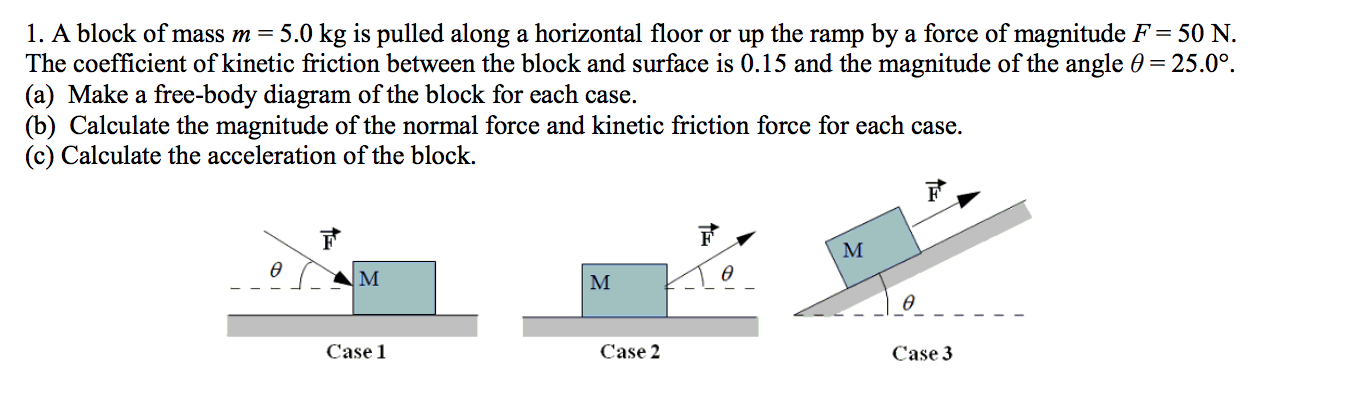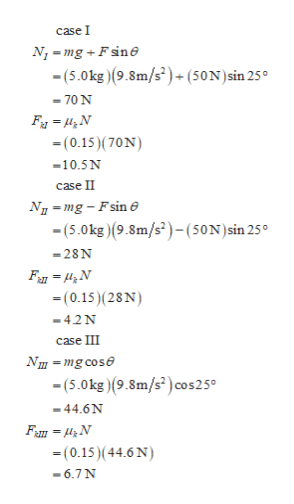# 1. A block of mass m = 5.0 kg is pulled along a horizontal floor or up the ramp by a force of magnitude F = 50 NThe coefficient of kinetic friction between the block and surface is 0.15 and the magnitude of the angle 0 25.0°.(a) Make a free-body diagram of the block for each case(b) Calculate the magnitude of the normal force and kinetic friction force for each case(c) Calculate the acceleration of the block.FМөө|мөCase 1Case 2Case 3

Question
22 viewshelp_outlineImage Transcriptionclose1. A block of mass m = 5.0 kg is pulled along a horizontal floor or up the ramp by a force of magnitude F = 50 N The coefficient of kinetic friction between the block and surface is 0.15 and the magnitude of the angle 0 25.0°. (a) Make a free-body diagram of the block for each case (b) Calculate the magnitude of the normal force and kinetic friction force for each case (c) Calculate the acceleration of the block. F М ө ө |м ө Case 1 Case 2 Case 3 fullscreen
check_circle

Step 1

(a)The free body diagram of each  mass are shown below.

Step 2

(b)For  case I,II, and III  the nor...help_outlineImage Transcriptionclosecase I N-mgFsin (5.0kg)(9.8m/s2)+ (50N) sin 25 70 N (0.15)(70N) 10.5N case II NI-mg-Fsin e (5.0kg)(9.8m/s2)-(50N) sin 25° -28N (0.15) (28N) -42N case III (5.0kg)(9.8m/s) cos2 5° -44.6N (0.15)(44.6 N) 6.7 N fullscreen

### Want to see the full answer?

See Solution

#### Want to see this answer and more?

Solutions are written by subject experts who are available 24/7. Questions are typically answered within 1 hour.*

See Solution
*Response times may vary by subject and question.
Tagged in

### Kinematics